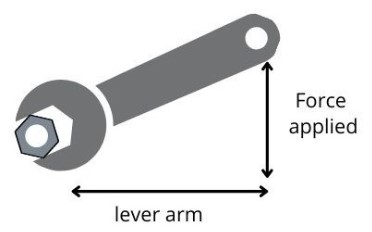# Moment of force calculator

Enter Force and Lever arm length in the moment of force calculator to find the moment of force.

N
m

Give Us Feedback

This moment arm calculator calculates the force around an axis (torque) aka moment of force. It simply uses lever arm length and force applied to find the moment of force.

You can find any of the three mentioned values.

## What is the moment of force?

Also known as torque, a moment of force is a force around a pivot point. It Is produced by force and distance from the pivot point.Torque is produced when a rigid body fixed at a point, on applying force,  moves in a circular motion about that point. Its SI unit is Nm

## Torque formula

Torque is calculated using this formula.

τ = rF

Where,

r = lever arm

F = force

## How to calculate the moment of force?

Example

A mechanic is loosening a nut with a crescent wrench of a lever arm 2 meters. The force applied is 5 Newtons. Calculate the moment of arm?

Solution:

Step 1: Identify the values in the given problem.

r = 2 m

f = 5 N

Step 2: Multiply both values.

T = r x F

T = 2 x 5

T = 10 Nm

### Math Tools